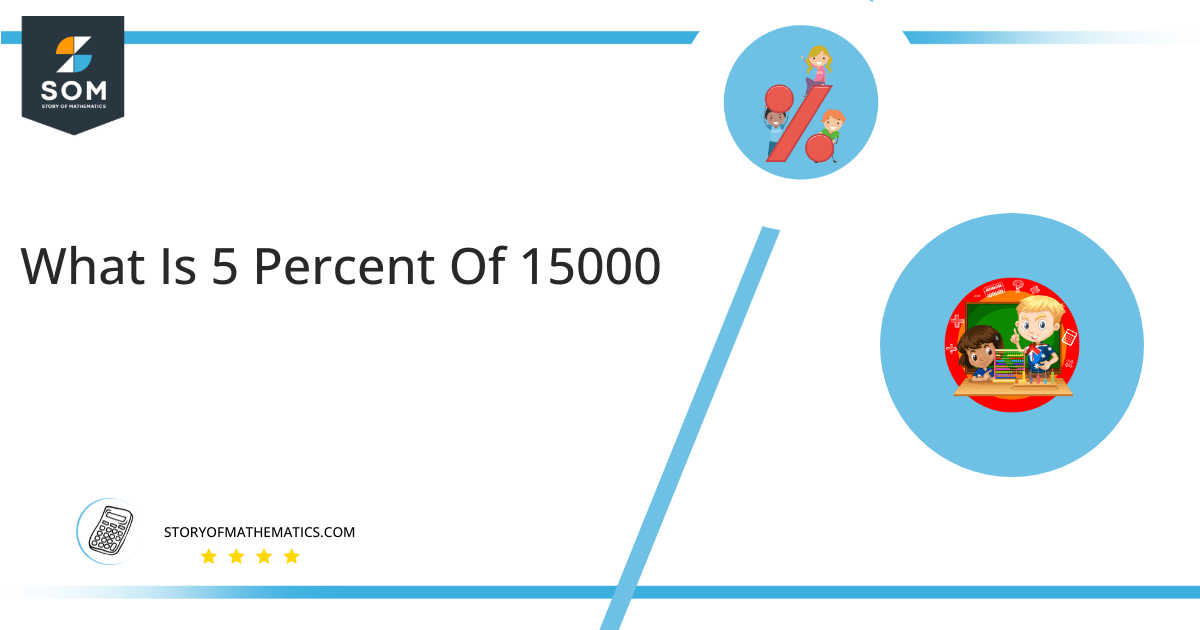# What Is 5 Percent of 15000 + Solution with Free Steps?The 5 percent of 15000 is equal to 750. It can be easily calculated by dividing 5 by 100 and multiplying the answer with 15000 to get 750.

The easiest way to get this answer is by solving a simple mathematical problem of percentage. You need to find 5% of 15000 for some sale or real-life problem. Divide 5 by 100, multiply the answer with 15000, and get the 5% of 15000 value in seconds.

This article will explain the full process of finding any percentage value from any given quantity or number with easy and simple steps.

## What Is 5 percent of 15000?

The 5 percent of 15000 is 750.

The percentage can be understood with a simple explanation. Take 15000, and divide it into 100 equal parts. The 5 number of parts from the total 100 parts is called 5 percent, which is 750 in this example.

## How To Calculate 5 percent of 15000?

You can find 5 percent of 15000 by some simple mathematical steps explained below.### Step 1

Firstly, depict 5 percent of 15000 as a fractional multiple as shown below:

5% x 15000

### Step 2

The percentage sign % means percent, equivalent to the fraction of 1/100.

Substituting this value in the above formula:

= (5/100) x 15000

### Step 3

Using the algebraic simplification process, we can arithmetically manipulate the above equation as follows:

= (5 x 15000) / 100

= 75000 / 100

= 750This percentage can be represented on a pie chart for visualization. Let us suppose that the whole pie chart represents the 15000 value. Now, we find 5 percent of 15000, which is 750. The area occupied by the 750 value will represent the 5 percent of the total 15000 value. The remaining region of the pie chart will represent 95 percent of the total 15000 value. The 100% of 15000 will cover the whole pie chart as 15000 is the total value.

Any given number or quantity can be represented in percentages to better understand the total quantity. The percentage can be considered a quantity that divides any number into hundred equal parts for better representation of large numbers and understanding.

Percentage scaling or normalization is a very simple and convenient method of representing numbers in relative terms. Such notations find wide application in many industrial sectors where the relative proportions are used.